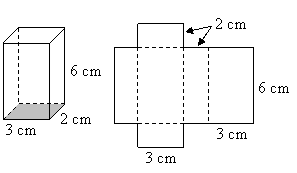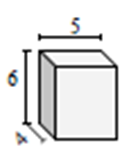# Using a net to find the surface area of a rectangular prism

In this lesson, we use nets of a solid figure to find the surface area of a solid figure.

The net of a solid figure is formed when a solid figure is unfolded along its edges and its faces are laid out in a pattern in two dimensions.

Nets of rectangular prisms are made up of rectangles and squares.

Using a net to find the surface area of a rectangular prism

Finding the areas of each of the rectangles and squares of the net of a rectangular prism and adding up those areas gives the surface area or total surface area of the prism.

For example, if the length of one side of the cube 4 units then the area of one its face is 4 × 4 = 16 square units. From the net, we can see that there are six equal faces and so we get the total surface area is 6 × 16 = 96 square units.

Surface Area of a Rectangular Prism using Nets

A rectangular prism or cuboid is formed by folding a net as shown −We can see from the net that there are two rectangles with dimensions 3 cm by 6 cm, two rectangles with dimensions 2 cm by 6 cm and two rectangles with dimensions 2 cm by 3 cm. The total surface area is then

2 × 3 × 6 + 2 × 2 × 6 + 2 × 2 × 3 = 72 cm2

Find the surface area of the given rectangular prism in square cm.### Solution

Step 1:

Using net, the surface area of a rectangular prism

= 2(l w + w h + l h); l = 6 ; w = 5; h = 3

Step 2:

Surface area of given prism = 2(6 × 5 + 6 × 3 + 3 × 5)

= 2(30 + 18 + 15)

= 126 square cm

Find the surface area of the given rectangular prism in square cm.### Solution

Step 1:

Using net, the surface area of a rectangular prism

= 2(l w + w h + l h); l = 5; w = 6; h = 4

Step 2:

Surface area of given prism = 2(5 × 6 + 5 × 4 + 6 × 4)

= 2(30 + 20 + 24)

= 148 square cm